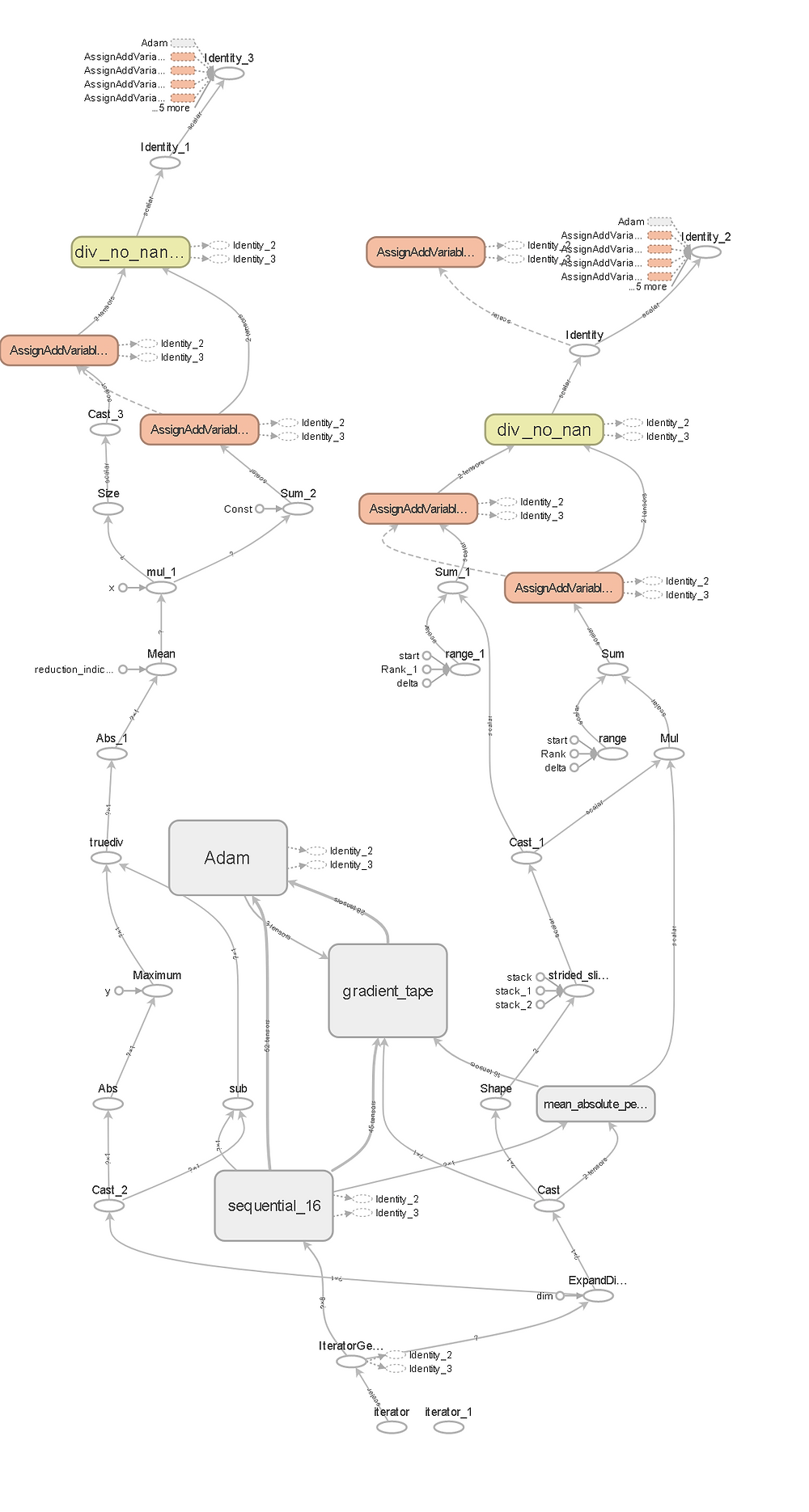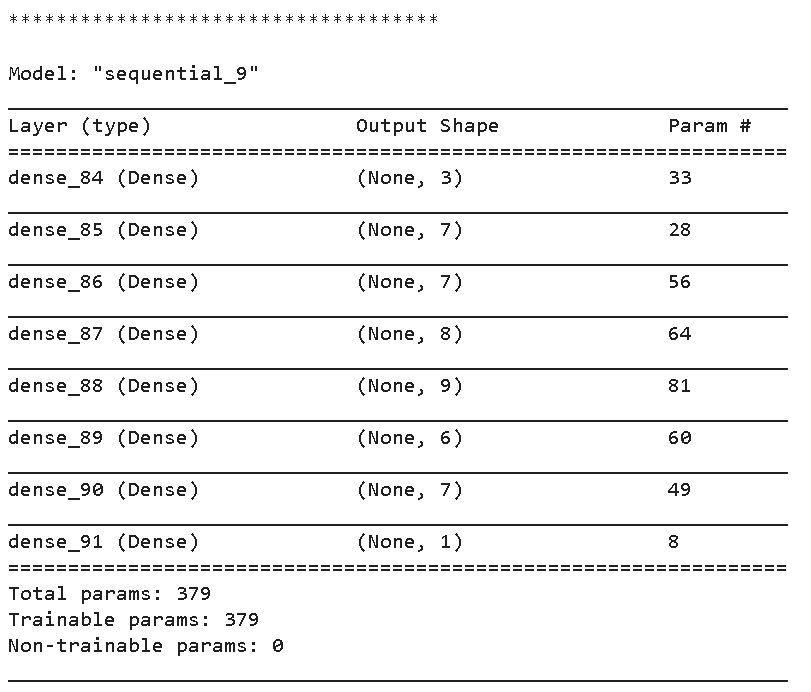Search

# Deep Neural Network: Left to Chance

Designing the architecture of a deep neural network is difficult. There are multiple decisions to be made regarding the network architecture: layers, depth, width, activations, initializations, regularizations... The world of deep neural networks is vast, very technically complex, and very difficult to understand completely. When faced with the necessity to explore the usage of a deep neural network (DNN for short), the challenge of selecting an initial configuration is daunting.

This is why we will not try to understand everything in full and simultaneously, with time constraints in mind, we are going to leave to chance as many of the architectural parameters of a DNN. We assume that we are so ignorant and the topic so broad that a computer typing randomly will find a good architecture faster than us.

We will use Tensorflow with Keras to generate a DNN that will be able to produce a value for a regression problem. A full model diagram, as displayed using Tensorboard, looks like this:The full model is quite complex, and the architecture of the DNN is in the nondescript "sequential_16" box, which contains this:This is a sequential model composed of dense neural network layers. Each layer also contains multiple parameters and those we are going to randomize. First, we are going to check what Keras has to offer us in terms of neuron layer activation and initialization:

```import keras as keras
I = keras.initializers.__dict__.items()
A = keras.activations.__dict__.items()

initializers = [name for name, cls in I if isinstance(cls, type)]

activations = [name for name, cls in A if callable(cls) and name.islower()]

print(initializers)
print(activations)```

From the Keras modules, we take the dictionary items that describe them, then for initializers, we take all the class instances, and for activations, we take all functions (are "callable") whose name starts with a lower case character:

```['ConstantV2', 'GlorotNormalV2', 'GlorotUniformV2', 'HeNormalV2', 'HeUniformV2', 'IdentityV2', 'LecunNormalV2', 'LecunUniformV2', 'OnesV2', 'OrthogonalV2', 'RandomNormalV2', 'RandomUniformV2', 'TruncatedNormalV2', 'VarianceScalingV2', 'ZerosV2', 'glorot_normalV2', 'glorot_uniformV2', 'he_normalV2', 'he_uniformV2', 'lecun_normalV2', 'lecun_uniformV2', 'Constant', 'constant', 'GlorotNormal', 'glorot_normal', 'GlorotUniform', 'glorot_uniform', 'HeNormal', 'he_normal', 'HeUniform', 'he_uniform', 'Identity', 'identity', 'Initializer', 'initializer', 'LecunNormal', 'lecun_normal', 'LecunUniform', 'lecun_uniform', 'Ones', 'ones', 'Orthogonal', 'orthogonal', 'RandomNormal', 'random_normal', 'RandomUniform', 'random_uniform', 'TruncatedNormal', 'truncated_normal', 'VarianceScaling', 'variance_scaling', 'Zeros', 'zeros', 'normal', 'uniform', 'one', 'zero']

['deserialize_keras_object', 'serialize_keras_object', 'keras_export', 'softmax', 'elu', 'selu', 'softplus', 'softsign', 'swish', 'relu', 'gelu', 'tanh', 'sigmoid', 'exponential', 'hard_sigmoid', 'linear', 'serialize', 'deserialize', 'get']```

We still have some unwelcome guests inside the initializers and activations list. We need to get rid of serialization activations in our regression model, all initializers starting with upper case character and some elements that are not activations or initializers:

```remove = ['get', 'keras_export', 'get_globals', 'initializer']

activations = [a for a in activations if 'serial' not in a]
activations = [a for a in activations if a not in remove]

initializers = [init for init in initializers if init.islower() ]
initializers = [init for init in initializers if init not in remove]

print(initializers)
print(activations)```

Now we can define a randomized neural network using these initialization and activation components. But, first, we will bound the number of input features and the width and height of the network to avoid giant monstrosities from occurring:

```n_features = N = 10
max_depth = D = 10
max_width = W =10```

To build our random network, we define this function:

```import random
from keras.models import Sequential
from keras.layers import Dense

def make_random_model(N, D, W):
model = Sequential()

# The Input Layer:
init = random.choice(initializers)
act = random.choice(activations)
width = random.randint(1, W)
input_dim=N, kernel_initializer=init))

# The Hidden Layers:
layers = random.randint(1, max_depth)
for i in range(layers):
init = random.choice(initializers)
act = random.choice(activations)
width = random.randint(1, W)

# The Output Layer:
init = random.choice(initializers)
return model```

With Sequential(), we are starting the definition of a model. We randomly choose the initialization, the activation, and the width for the input layer: the number of dense neurons in the layer. The input layers' input dimensions must be equal to the number of features of our regression problem. For the hidden layers, we first decide on several layers between 1 and our maximum depth, then for each of these layers, we repeat the initialization, activation, and width choices. Finally, the output layer must contain a single dense neuron if we regress for a single value, with a linear activation and a random initializer.

Activations and initializers are complex subjects, and unless we understand them all fully, we may be making an erroneous choice for a given model. However, we do not care anymore as our computer will generate multiple models randomly, and it does not know hyperbolic tangents from rectifier units:

```make_n = 20
for _ in range(make_n):
random_model = make_random_model(N, D, W)
random_model.summary()
print('\n************************************\n')```

The computer gifts us with 20 newly minted, probably useless, neural network models like this one:Is this particular one useful? We do not know. We will need to bring it to some tests, using regression problem data, and see if the random generator can generate models that can do regression. We will test a myriad of random networks in our next post.

If you require quantitative model development, deployment, verification, or validation, do not hesitate and contact us. We will also be glad to help you with your machine learning or artificial intelligence challenges when applied to asset management, automation, or intelligence gathering from satellite, drone, or fixed-point imagery.

The notebook, in Google Colab, for this post is located here.